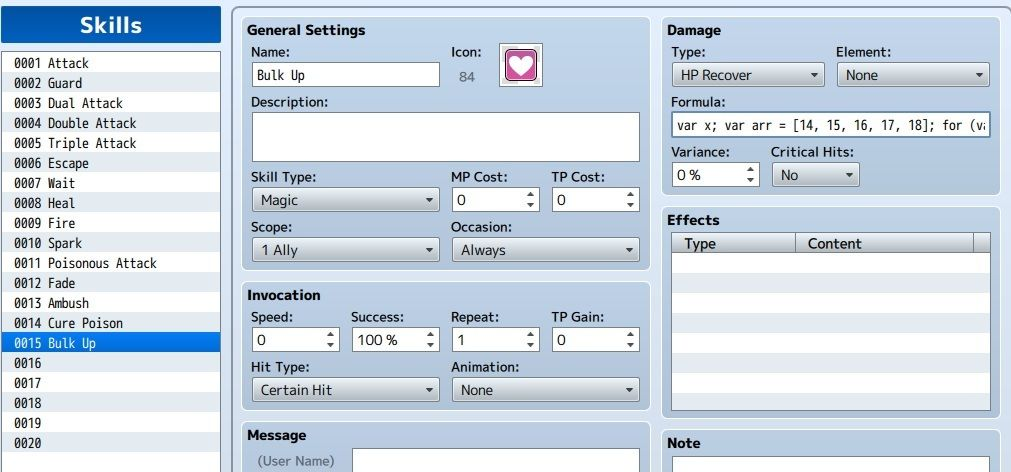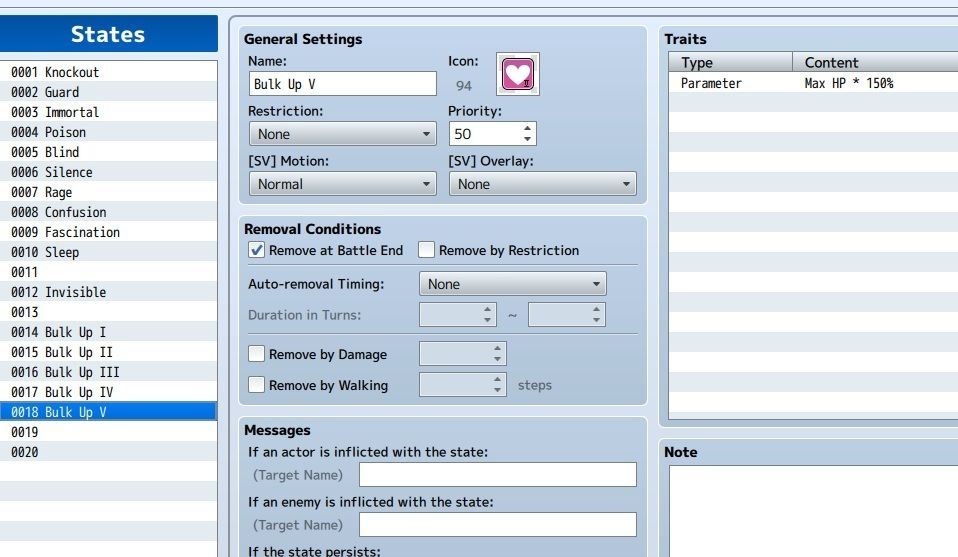# RMMV Damage Formula - ideas and help

#### luigiman2201

##### VillagerI can't wait for there to be a better explanation of the formulaic system, such as what is and isn't allowed, or what terms do what. For instance, I sat here and tried to figure out a Huskar-esque (DOTA2 character) attack would look like in terms of a.atk * 4 + (a.hp {divided? no idea what sign you use}/2) - .... Ok, so basically what I'm trying to say is user's attack times four (like a normal attack) plus the user's current hp divided by two, subracted by the regular physical attack defense.

Not so sure how to achieve that... Or what all the terms are.
Maybe this will do it:

a.atk * 4 + (a.hp / 2) - b.defOtherwise, you could always multiply the a.hp by 0,5

Last edited by a moderator:

#### Zarsla

##### VeteranI can't wait for there to be a better explanation of the formulaic system, such as what is and isn't allowed, or what terms do what. For instance, I sat here and tried to figure out a Huskar-esque (DOTA2 character) attack would look like in terms of a.atk * 4 + (a.hp {divided? no idea what sign you use}/2) - .... Ok, so basically what I'm trying to say is user's attack times four (like a normal attack) plus the user's current hp divided by two, subracted by the regular physical attack defense.

Not so sure how to achieve that... Or what all the terms are.
terms:

a=user's stats

b=target's stats

atk = physical attack

def = defense

mat = magical attak

mdf = magical defense

luk = luck

agi = agility

Eg Harold (the user) atacks a slime (the target) using the basic forumula attack skill, the forumula, a.atk * 4 - b.def * 2, is intterupted like this:

the slimes defense times 2 taken away from 4 times harolds attack. (The forumulas work like genric order if operations, so multiplacation and division are done first then subtraction and divison. )

Last edited by a moderator:

#### MikeMakes

##### VeteranIs there a syntax for: adding states 2 through 5?

#### RockEsper

##### VillagerIn your original post you said you wanted them to do 1x damage only if they had at least 10 luck, so mine accommodates for that
Mine doesn't accommodate for that?

#### VHStapes

##### Game DesignerMine doesn't accommodate for that?
EDIT: You're right it does, I was getting myself all confused.

Last edited by a moderator:

#### Shaz

##### VeteranIs there a syntax for: adding states 2 through 5?
Code:
``for (i = 2; i <= 5; i++) { b.addState(i) }``

•MikeMakes

#### MikeMakes

##### VeteranThank you, Shaz. It works perfectly. I didn't thought the syntax would be so long though. But good thing that the formula box can handle it.

#### Xekin

##### WarperHello, I'm looking for a formula that increases the damage of the skill based on how many party members are dead, I tried with this formula: \$gameParty.deadMembers() * 100 and different variables, but it makes 0 damage when no party members are dead and No damage when 1 or more are dead. Any help?

Thanks!

#### WarpBanshee

##### VillagerCan anyone help me fix my formula?

c = Math.randomInt(20) + a.atk; d = Math.randomInt(20) + b.def; if c> d; c=a.matk; else c=0; end;

I'm trying to get a random number between 1-20 to essentially be "rolled" for both the attacker's ATK stat and the defender's defense stat, and if the ATK + roll is greater to do damage based on the attacker's MATK or else do nothing. Currently, whenever anyone uses the formula for this skill it always generates 0's.

#### Shaz

##### VeteranTry this:

(Math.randomInt(20) + a.atk) > (Math.randomInt(20) + b.def) ? a.matk : 0

I think the issue is that you're using lots of ; instead of {} in your if/then/else test. Also, 0 is what's returned if you have an error in your formula.

The above removes the need for the temporary variables and makes it shorter.

Note that Math.randomInt gives a number between 0 and 19, not between 1 and 20, but for these purposes it doesn't really matter, as it's doing it for both sides.

#### Sinweaver

##### VeteranTrying to work my way around the lack of stackable state with the damage formula bar..

So far, it seems to work, but in a way it is not supposed to.

var x; var arr = [14, 15, 16, 17, 18]; for (var i = 0, len = arr.length; i < len; i++){x = b.isStateAffected(arr) ? arr[i+1] : arr}; b.addState(x); x;Note: the x at the end is used for testing purpose.

What I wanted to do was to apply a HP bonus buff that can be stack for up to 5 times.

I store the 5 states in an array called arr

var arr = [14, 15, 16, 17, 18];Then I put it through a loop to see if the target is affected by state arr

for (var i = 0, len = arr.length; i < len; i++){x = b.isStateAffected(arr) ? arr[i+1] : arr};If the target is affected by state id 14, then arr[0+1] (state id 15) will be assigned to the variable x.

If the target is not affected by state id 14, then arr (state id 14) will be assigned to the variable x

b.addState(x);Apply state x to the target, the value for x is calculated in the loop.

When the skill is used in a test battle, it went ahead and apply state 18 (the highest state) instead of starting with 14 (the lowest) and slowly building it up.

I understand that this is the result of putting it through the loop, but is there a way to arrange the formula so that it exits the loop once it found the right state to apply?

I wanted to remove the lower ranked state whenever we repeat the use of the skill, except for rank 5, which will not be removed. But I guess I can figure this out once later.

Skill set up:State set up:Last edited by a moderator:

#### dayhjawk

##### VeteranI was wondering and hopefully this is the right place to ask.

Is it possible to setup a variable that would store a # that can be used inside the formula.

Example:

m.att - m.def * 0.33 * 1 (this "1" would be the variable number, that can be changed?)

#### Tobbx

##### VeteranI only want one of my Classes to gain TP for using the attack command. So i figured i use something like this:

a.gainTp(Math.randomInt(12)+1; Damage FormulaThe thing i'm wondering is if it's possible to make an IF statement before the TP formula to check if the class of the attacker is equal to Class ID 1. if (Condition) { a.gainTp(Math.randomInt(12)+1}; But then the damage would be ignored also. So i guess i have to write it like if (Condition) a.gainTp(Math.randomInt(12))+1; Damage Formula else; Damage Formula. You probably get what i want to do. But i don't know how to set it up properly.

Last edited by a moderator:

#### Sinweaver

##### VeteranI was wondering and hopefully this is the right place to ask.

Is it possible to setup a variable that would store a # that can be used inside the formula.

Example:

m.att - m.def * 0.33 * 1 (this "1" would be the variable number, that can be changed?)
\$gameVariables.value(n)

where n is the variable you are using

I only want one of my Classes to gain TP for using the attack command. So i figured i use something like this:

a.gainTp(Math.randomInt(12)+1; Damage Formula
The thing i'm wondering is if it's possible to make an IF statement before the TP formula to check if the class of the attacker is equal to Class ID 1. if (Condition) { a.gainTp(Math.randomInt(12)+1}; But then the damage would be ignored also. So i guess i have to write it like if (Condition) a.gainTp(Math.randomInt(12))+1; Damage Formula elseDamage Formula. You probably get what i want to do. But i don't know how to set it up properly.

Use this to check for class ID

a._classId === n ?

Replace n with the ID for the class

Last edited by a moderator:

#### Merancapeman

##### Veteranterms:

a=user's stats

b=target's stats

atk = physical attack

def = defense

mat = magical attak

mdf = magical defense

luk = luck

agi = agility
I meant what kind of equations can be done and their sign. I wasn't sure if "/" worked as division or what, but according to another reply it seems that's the case.

#### dayhjawk

##### VeteranWant to make sure this is working correctly:

\$gameTemp.reserveCommonEvent(8); a.mat - b.mdf * 0.33 * \$gameVariables.value(4)

the common event runs to see if the gamevariables.value(4) is either 1 or 2, then changes based on the event.

I am hoping my train of thought works.

#### Alucart

##### VillagerI want make a skill that absorbs HP or MP of certain percentage of the skill's damage...

do you guys have any idea what should I do?

#### Kenen

##### VeteranDo we know if there's a way to bypass the damage popup if a condition is met in the formula? E.g. something like...

Code:
``if (b.isStateAffected(86)) { b.result().used = false; }``

Last edited by a moderator:

#### luigiman2201

##### VillagerI want make a skill that absorbs HP or MP of certain percentage of the skill's damage...

do you guys have any idea what should I do?

before the actual damage formula, you can put in:

a.gainHp((damage formula)*percentage);damage formula

For example, if you're using the standard damage formula of

a.atk * 4 - b.def * 2and you absorb 25% of the damage, your formula becomes

a.gainHp((a.atk * 4 - b.def * 2) * 0,25);a.atk * 4 - b.def * 2If you want to absorb MP, use a.gainMp instead of a.gainHp

Let me know if there's anything you don't understand, and i'll try to explain.

•Daemien

#### dayhjawk

##### Veteranthis isn't a damage formula, but it is a formula related question.

i want to setup a formula that heals the target, and half back to the user of the skill. so if I heal a target for lets say 10 hit points, the user would get 5 back.

Last edited by a moderator:

### Latest Profile Posts

"If the lion knew her own strength, hard were it for anyone to rule her". This would be my custom title if I had enough spaces for it. Coming off of personal pain, I'm glad I found the original quote I've tweaked.
Today I found out that ladybirds\ladybugs are apparently named for the Virgin Mary. They're also known as God's cows in some places.
Well xD
The sad reaction was not because I want you to credit me. (terms clearly say no need to credit) but because those maps are meant to be used as inspiration or guidence. You are ofcourse alowed to use them however you want